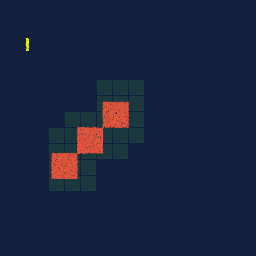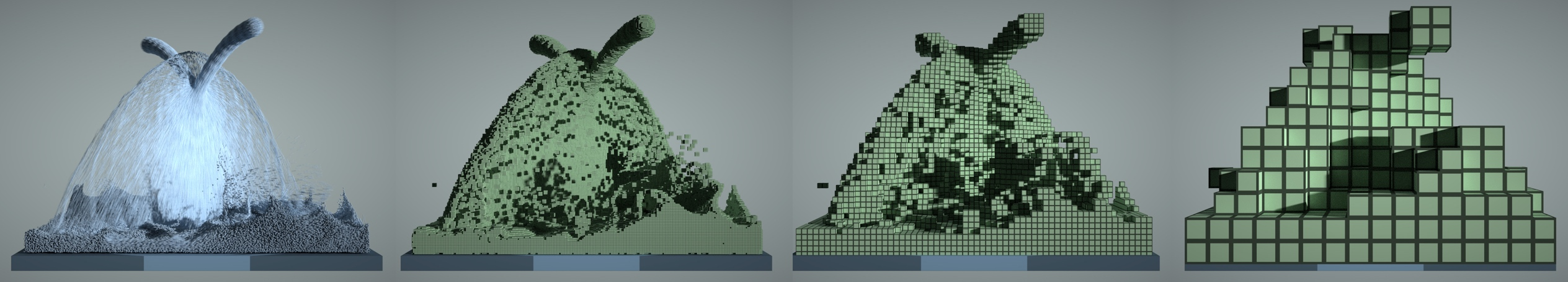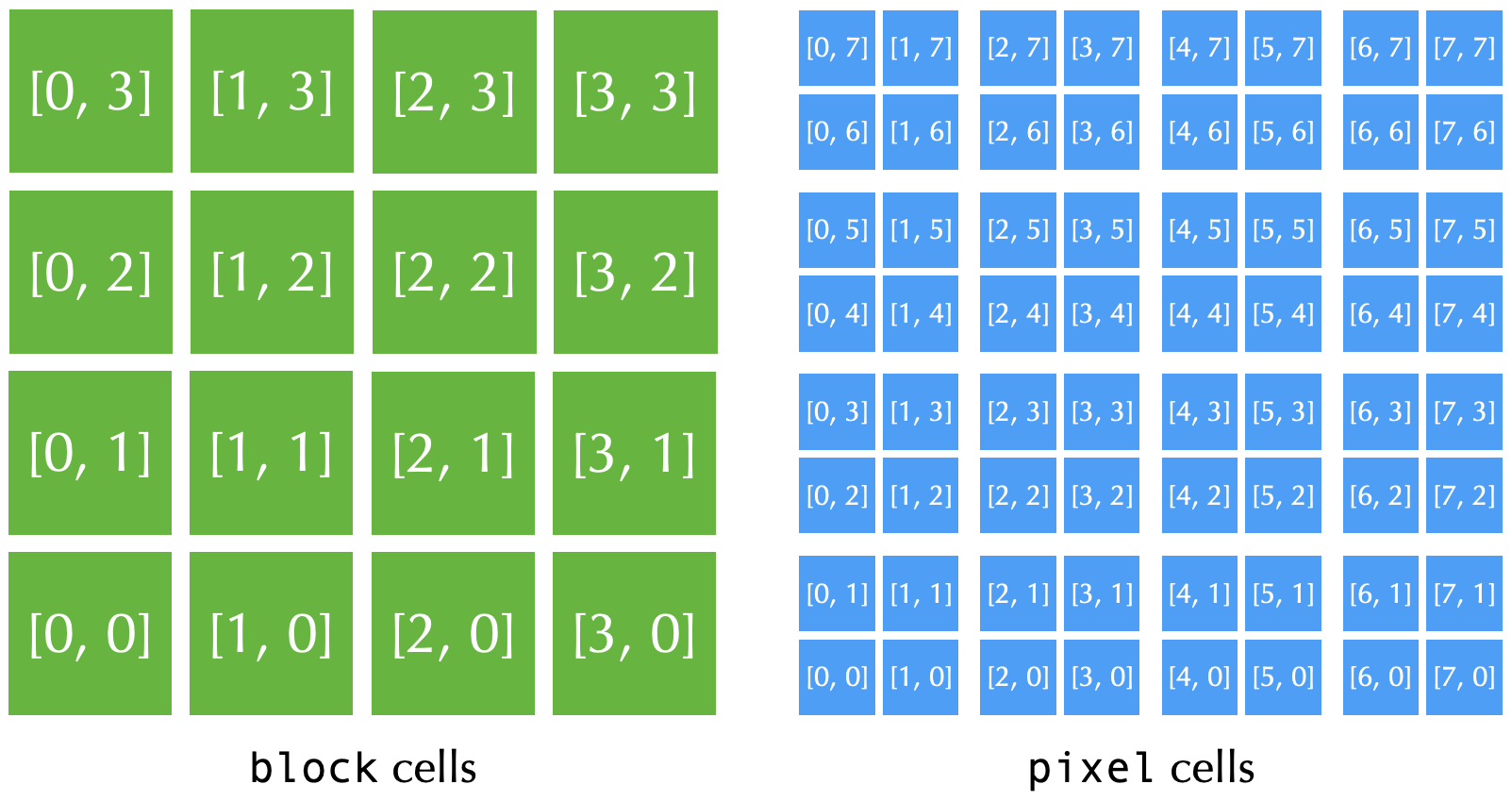Sparse computation

Compiler-level support for spatially sparse computation is a unique feature of Taichi.Figure: A swinging "Taichi" pattern represented with a 512x512 sparse grid. The sparse grid has a multi-level tree structure. White stands for inactive tree nodes, and active tree nodes are darker.

The sparse grid above has the following structure:

• The grid is divided into 8x8 block1 containers;
• Each block1 container has 4x4 block2 cells;
• Each block2 container has 4x4 block3 cells;
• Each block3 container has 4x4 pixel cells;
• Each pixel contains an i32 value x[i, j].
note

Taichi allows you to define sparse data structures effortlessly. For example, you can define the grid above as

x = ti.field(dtype=ti.i32)
block1 = ti.root.pointer(ti.ij, 8)block2 = block1.pointer(ti.ij, 4)block3 = block2.pointer(ti.ij, 4)block3.dense(ti.ij, 4).place(x)

[Full source code of this animation]

Intuitively, a sparse grid in Taichi allows you to use memory space more wisely, since only tree nodes involved in computation are allocated. Now, let's take a step back and think about why we need sparse grids, how to define them in Taichi, and how to compute on these data structures.

Motivation#

High-resolution 2D/3D grids are often needed in large-scale spatial computation, such as physical simulation, rendering, and 3D reconstruction. However, these grids tend to consume a huge amount of memory space and computation. While a programmer may allocate large dense grids to store spatial data (especially physical quantities such as a density or velocity field), oftentimes, they only care about a small fraction of this dense grid since the rest may be empty space (vacuum or air).

In short, the regions of interest in sparse grids may only occupy a small fraction of the whole bounding box. If we can leverage such "spatial sparsity" and focus computation on the regions we care about, we will significantly save storage and computing power.

note

The key to leverage spatial sparsity is to replace dense grids with sparse grids.

On a sparse data structure, we consider a pixel, voxel, or a grid node to be active, if it is allocated and involved in computation. The rest of the grid is simply inactive. The activity of a leaf or intermediate cell is a boolean value. The activity value of a cell is True if and only if the cell is active.

Below is a 2D multi-physics simulation (material point method) with 256x256 grid cells. Since the simulated objects do not fully occupy the whole domain, we would like to adaptively allocate the underlying simulation grid. We subdivide the whole simulation domain into 16x16 blocks, and each block has 16x16 grid cells. Memory allocation can then happen at block granularity, and we only consume memory space of blocks that are actually in the simulation.(Note the changing distribution of active blocks throughout the simulation.)

note

Backend compatibility: The LLVM backends (CPU/CUDA) and the Metal backend offer the full functionality of sparse computation. Other backends provide no or limited support of sparse computation.

note

Sparse matrices are usually not implemented in Taichi via (spatially-) sparse data structures. Use ti.SparseMatrixBuilder instead.

Defining sparse data structures in Taichi#

Ideally, it would be nice to have a sparse voxel data structure that consumes space or computation only when the voxels are active. Practically, Taichi programmers use hierarchical data structures (trees) to organize sparse voxel data.

Data structure hierarchy#

Traditionally, Quadtrees (2D) and Octrees (3D) are often adopted. Since dereferencing pointers is relatively costly on modern computer architectures, compared to quadtrees and octrees, it is more performance-friendly to use shallower trees with larger branching factors. VDB and SPGrid are such examples. In Taichi, programmers can compose data structures similar to VDB and SPGrid with SNodes.Figure: A 3D fluid simulation that uses both particles and grids. Left to right: particles, 1x1x1 voxels, 4x4x4 blocks, 16x16x16 blocks.

While a null pointer can effectively represent an empty sub-tree, at the leaf level using 64 bits to represent the activity of a single voxel can consume too much space. For example, if each voxel contains a single f32 value (4 bytes), the 64-bit pointer pointing to the value would take 8 bytes. The fact that storage costs of pointers are higher than the space to store the value themselves goes against our goal to use sparse data structures to save space.

To amortize the storage cost of pointers, programmers usually organize voxels in a blocked manner and let the pointers directly point to the blocks (instead of voxels).

One caveat of this design is that voxels in the same dense block can no longer change their activity flexibly. Instead, they share a single activity flag. To address this issue, the bitmasked SNode additionally allocates 1-bit per voxel data to represent the voxel activity.

A typical sparse data structure#

Sparse data structures in Taichi are usually composed of pointer, dense, and bitmasked SNodes. The code snippet below creates an 8x8 sparse grid, with the top level being 4x4 pointer arrays, and each pointer points to a 2x2 dense block.

x = ti.field(dtype=ti.i32)
block = ti.root.pointer(ti.ij, (4, 4))pixel = block.dense(ti.ij, (2, 2))pixel.place(x)Computation on sparse data structures#

Activation on write#

When writing to an inactive cell on a sparse data structure, Taichi automatically populates the data structure.

For example, when executing x[2, 3] = 2 on the aforementioned sparse grid x, Taichi automatically activates block[1, 1] so that pixel[2, 3] is allocated.

note

Reading an inactive voxel returns zero.

Sparse struct-fors#

Efficiently looping over sparse grid cells that distribute irregularly can be challenging, especially on parallel devices such as GPUs. In Taichi, struct-for's natively support sparse data structures and only loops over currently active voxels. The Taichi system ensures efficient parallelization. You can loop over different levels of the tree. The code below demonstrates the creation and manipulation of a sparse grid:

import taichi as ti
ti.init()
x = ti.field(dtype=ti.i32)block = ti.root.pointer(ti.ij, (4, 4))if use_bitmask:    pixel = block.bitmasked(ti.ij, (2, 2))else:    pixel = block.dense(ti.ij, (2, 2))pixel.place(x)
@ti.kerneldef sparse_struct_for():    x[2, 3] = 2    x[5, 6] = 3
for i, j in x:        print('field x[{}, {}] = {}'.format(i, j, x[i, j]))
for i, j in block:        print('Active block: [{}, {}]'.format(i, j))

When use_bitmask = True, the program above outputs

field x[2, 3] = 2field x[5, 6] = 3Active block: [1, 1]Active block: [2, 3]

When use_bitmask = False, we get

field x[2, 2] = 0field x[2, 3] = 2field x[3, 2] = 0field x[3, 3] = 0field x[4, 6] = 0field x[4, 7] = 0field x[5, 6] = 3field x[5, 7] = 0Active block: [1, 1]Active block: [2, 3]

When using a dense SNode as the leaf block, activating x[2, 3] also implicitly activates other pixels in block[1, 1], i.e., x[2, 2], x[3, 2], and x[3, 3]. Without a bitmask, these pixels in the same block share the same activity.

Explicitly manipulating and querying sparsity#

Taichi also provides APIs that explicitly manipulates data structure sparsity.

• Use ti.is_active(snode, [i, j, ...]) to query if snode[i, j, ...] is active or not.
• ti.activate/deactivate(snode, [i, j, ...]) to explicitly activate or deactivate a cell of snode[i, j, ...].
• Use snode.deactivate_all() to deactivate all cells of SNode snode. This operation also recursively deactivates all its children.
• Use ti.deactivate_all_snodes() to deactivate all cells of all SNodes with sparsity.
• Use ti.rescale_index(descendant_snode/field, ancestor_snode, index) to compute the ancestor index given a descendant index.

Below is an example of these APIs:

import taichi as ti
ti.init()
x = ti.field(dtype=ti.i32)block1 = ti.root.pointer(ti.ij, (4, 4))block2 = block1.pointer(ti.ij, (2, 2))pixel = block2.dense(ti.ij, (2, 2))pixel.place(x)
@ti.kerneldef sparse_api_demo():    ti.activate(block1, [0, 1])    ti.activate(block2, [1, 2])
for i, j in x:        print('field x[{}, {}] = {}'.format(i, j, x[i, j]))    # outputs:    # field x[2, 4] = 0    # field x[2, 5] = 0    # field x[3, 4] = 0    # field x[3, 5] = 0
for i, j in block2:        print('Active block2: [{}, {}]'.format(i, j))    # output: Active block2: [1, 2]
for i, j in block1:        print('Active block1: [{}, {}]'.format(i, j))    # output: Active block1: [0, 1]
for j in range(4):        print('Activity of block2[2, {}] = {}'.format(j, ti.is_active(block2, [1, j])))
ti.deactivate(block2, [1, 2])
for i, j in block2:        print('Active block2: [{}, {}]'.format(i, j))    # output: nothing
for i, j in block1:        print('Active block1: [{}, {}]'.format(i, j))    # output: Active block1: [0, 1]
print(ti.rescale_index(x, block1, ti.Vector([9, 17])))    # output = [2, 4]
# Note: ti.Vector is optional in ti.rescale_index.    print(ti.rescale_index(x, block1, [9, 17]))    # output = [2, 4]
ti.activate(block2, [1, 2])
sparse_api_demo()
@ti.kerneldef check_activity(snode: ti.template(), i: ti.i32, j: ti.i32):    print(ti.is_active(snode, [i, j]))
check_activity(block2, 1, 2) # output = 1block2.deactivate_all()check_activity(block2, 1, 2) # output = 0check_activity(block1, 0, 1) # output = 1ti.deactivate_all_snodes()check_activity(block1, 0, 1) # output = 0
note

For performance reasons, ti.activate(snode, index) only activates snode[index]. The programmer must ensure all ancestor containers of snode[index] are already active. Otherwise, this operation results in undefined behavior.

Similarly, ti.deactivate ...

• does not recursively deactivate all the descendants of a cell.
• does not trigger an deactivation of its parent container, even if all the children of the parent container are deactivated.
note

When deactivation happens, the Taichi runtime automatically recycles and zero-fills memory of the deactivated containers.

note

While it is possible to directly use [i // 2, j // 2] to compute the block index given pixel index, doing so couples computation code with the internal configuration of data structures (in this case, the size of block containers).

Use ti.rescale_index to avoid hard-coding internal information of data structures.